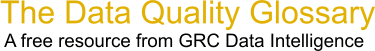Choose Index below for a list of all words and phrases defined in this glossary.

# Standard Deviation Calculation

index | Index

## Standard Deviation Calculation - definition(s)

Standard deviation calculation - A measure of dispersion of a frequency distribution that is the square root of the arithmetic mean of the squares of the derivation of each of the class frequencies from the arithmetic mean of the frequency distribution. Also a similar quantity found by dividing by one less than the number of squares in the sum of squares instead of taking the arithmetic mean.

[Category=Data Quality ]

Source: Larry English, http://www.iaidq.com/main/glossary.shtml, 10-Feb-2009 12:49

Data Quality Glossary.  A free resource from GRC Data Intelligence. For comments, questions or feedback: dqglossary@grcdi.nl